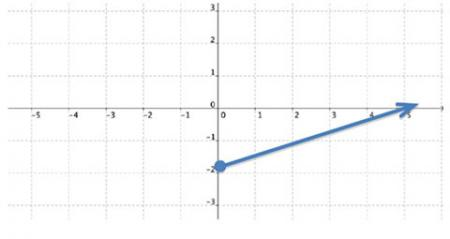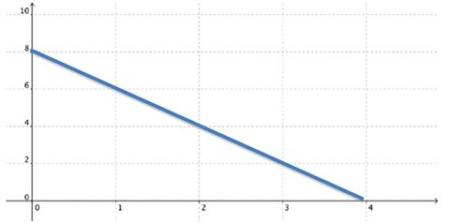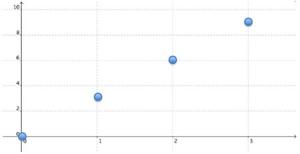# Let's Get Started

Given a real-world situation that can be modeled by a linear function or a graph of a linear function, determine and represent a reasonable domain and range of the linear function by using inequalities.

TEKS Standards and Student Expectations

A(2)(A) determine the domain and range of a linear function in mathematical problems; determine reasonable domain and range values for real‐world situations, both continuous and discrete; and represent domain and range using inequalities

Resource Objective(s)

Given a verbal statement or a graph of a linear function, determine its domain and range.

Essential Questions

What is domain?

What is range?

Which variable is the independent variable?

Which variable is the dependent variable?

Vocabulary

# Determining the Domain and Range Modeled by a Linear Function

To determine the domain of a given situation, identify all possible x-values, or values of the independent variable. To determine the range of a given situation, identify all possible y-values, or values of the dependent variable.

Example 1
A clown at a birthday party can blow up five balloons per minute. The relationship between the number of balloons inflated and the time that has passed can be expressed with the equation y = 5x, where x is the number of minutes passed and y is the number of balloons inflated. Find the domain and range of the relations.In this example, the independent variable (x) is the number of minutes. The possible x-values include all real numbers greater than or equal to 0, since time can be measured in fractional parts of a minute.

The dependent variable (y) is the number of balloons inflated. The possible y-values include all real numbers greater than or equal to 0.

Therefore, the domain is {x ≥ 0}, and the range is {y ≥ 0}.

# DVD Rental Example

An online DVD rental site charges a monthly membership fee of $10, plus$4 per DVD that is rented. The relationship between the number of DVDs rented and the total charge per month can be expressed with the equation y = 4x + 10, where x is the number of DVDs rented and y is the total charge per month. Find the domain and range of this relationship.

In this example, the independent variable, x, is the number of DVDs rented. The possible x-values include all whole numbers, since only whole number of DVDs can be rented.

The dependent variable, y, is the total charge per month. The possible y-values include 10, 14, 18, 22, . . .

Therefore, the domain is {0,1,2,3, . . .}, and the range is {10,14,18, 22, . . .}.

# Determining the Domain from a Graph

Identify the set of all the x-coordinates in a function’s graph to determine the domain.In this example, the domain is {≥ 0}, since 0 is the lowest x-value and the arrow indicates the line continues to the right. The boundary number of 0 is included, since the dot is solid.

Identify the set of all the y-coordinates in the function’s graph to determine the range.

In this example, the range is {≥ -2}, since -2 is the lowest y-value and the arrow indicates the line continues upward. The boundary number of -2 is included, since the dot is solid.

# Another Example# Activity 1: Graphit Domain and Range

Click on the following link to go to the interactive Graphit page.

• Enter the following functions into the y(x) box.  Click "Plot/Update" and view the resulting graphs.
• Record the domain and range for each function in your OnTRACK Algebra Journal.  Function Domain Range The cost to park in a garage is a $5 entry fee plus$2 per hour. y(x) = 2x + 5 The amount of snow that fell after midnight is 3 feet per hour. y(x) = 3x A swimming pool is draining at a rate of 1.5 feet per minute. y(x) = -1.5x